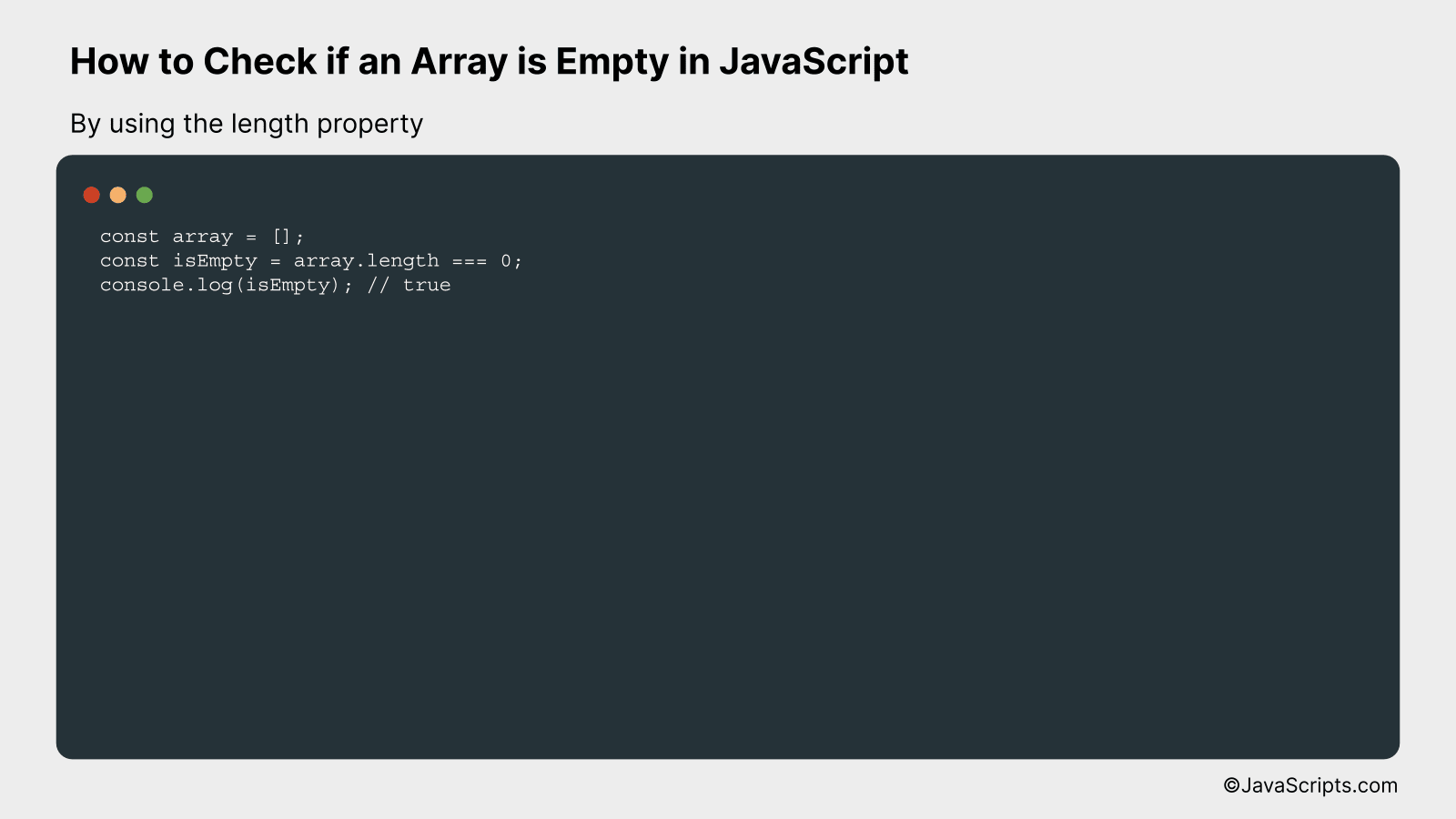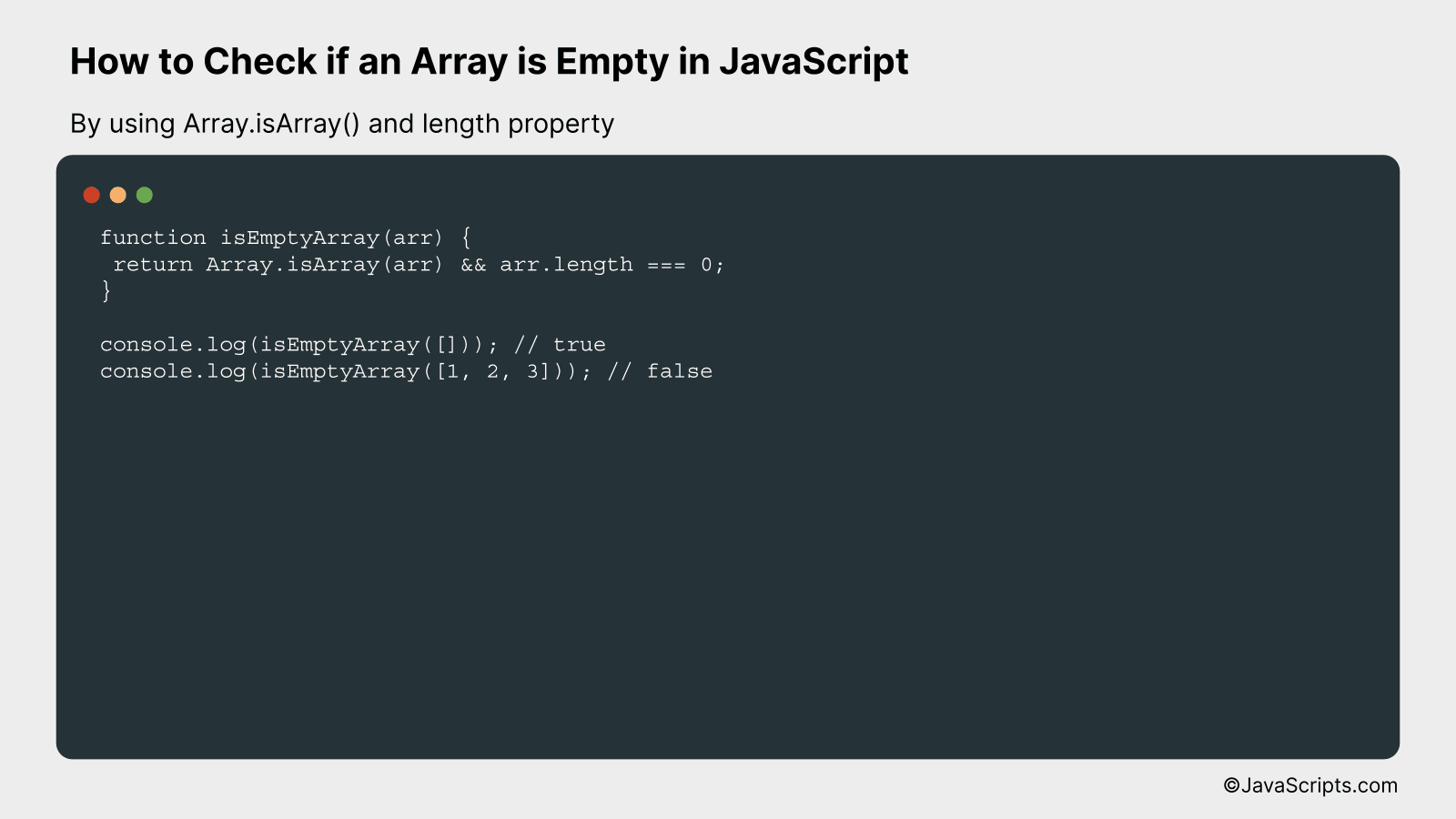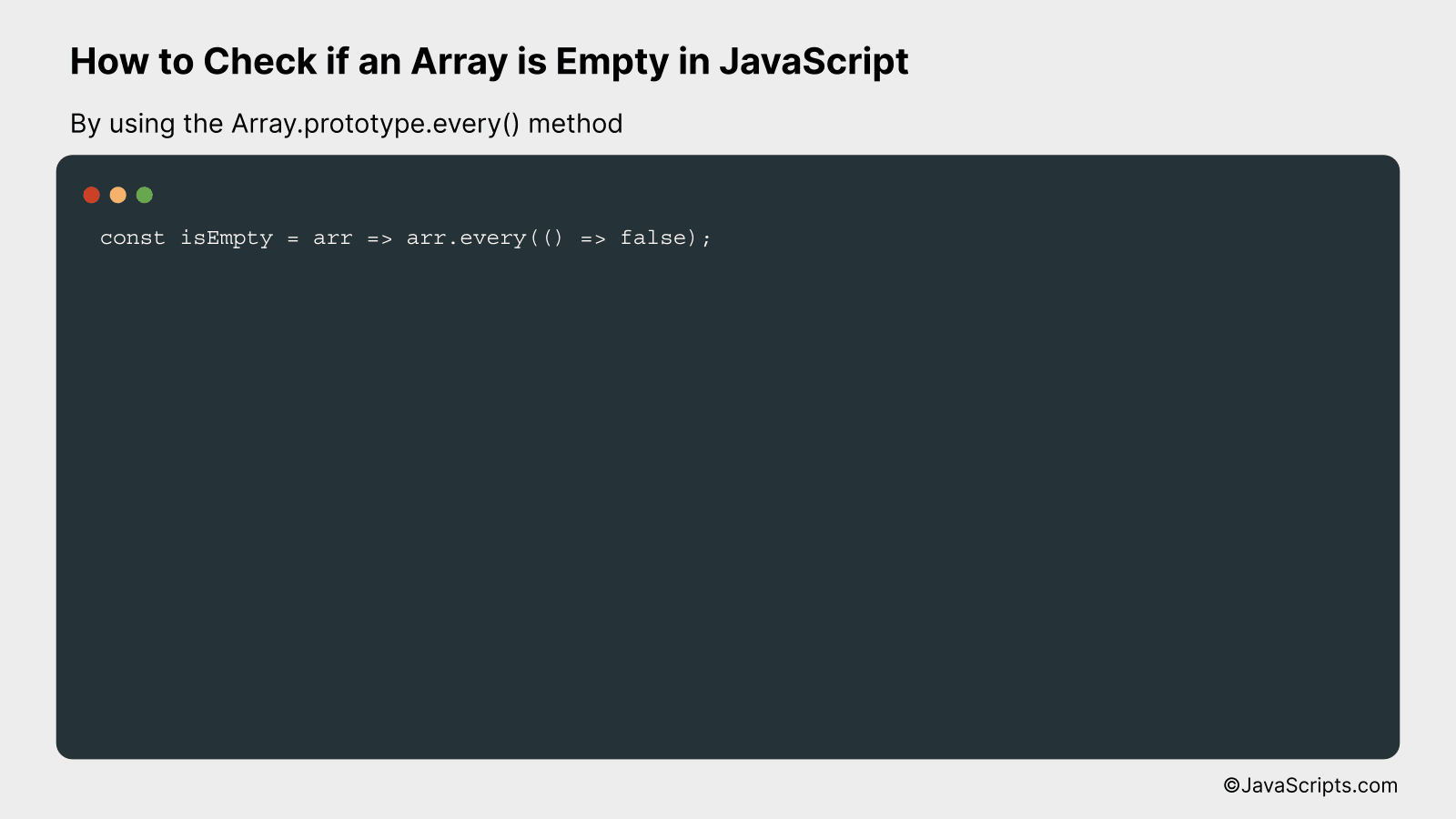# How to Check if an Array is Empty in JavaScript

When working with JavaScript, you’ll often find yourself dealing with arrays. These powerful data structures store multiple values in a single variable, making them indispensable for handling data. However, how do you ascertain when an array is empty?

Well, you’re in the right place to discover the answer. As we navigate through the world of coding together, we’ll unravel various methods to validate if an array has no elements. You’ll find, it’s simpler than you might think!

In this post, we will be looking at the following 3 ways to check if an array is empty in JavaScript:

• By using the length property
• By using Array.isArray() and length property
• By using the Array.prototype.every() method

Let’s explore each…

## #1 – By using the length propertyCheck if an array is empty in JavaScript using the length property, which returns the number of elements in the array. If the length is 0, the array is empty. Here’s an example:

``````
const array = [];
const isEmpty = array.length === 0;
console.log(isEmpty); // true
``````

### How it works

By using the length property on an array, you can check if it is empty or not. If the length is 0, it implies that there are no elements in the array, and it is empty.

• Create an array (e.g., `const array = [];`).
• Use the `length` property of the array to get the number of elements (e.g., `array.length`).
• Check if the length of the array is 0, which implies that the array is empty (e.g., `const isEmpty = array.length === 0;`).
• Print the value of `isEmpty` to the console (e.g., `console.log(isEmpty);`). If the result is `true`, the array is empty; otherwise, it is not.

## #2 – By using Array.isArray() and length propertyIn JavaScript, you can check if an array is empty by using the `Array.isArray()` method in combination with the `length` property. This approach ensures that the provided input is an array and has a length of zero. For example:

``````
function isEmptyArray(arr) {
return Array.isArray(arr) && arr.length === 0;
}

console.log(isEmptyArray([])); // true
console.log(isEmptyArray([1, 2, 3])); // false
``````

### How it works

The function `isEmptyArray()` checks if the provided input is an empty array by leveraging the `Array.isArray()` method and the `length` property. Here’s a step-by-step explanation of how it works:

• Step 1: Check if the input is an array using the `Array.isArray()` method. This returns `true` if the input is an array and `false` otherwise.
• Step 2: Check the input array’s length using the `length` property. If the length is 0, it means the array is empty.
• Step 3: Combine the results of the two checks using the AND operator (`&&`). The function returns `true` if both checks pass (i.e., the input is an array and its length is 0) and `false` otherwise.

## #3 – By using the Array.prototype.every() methodThe Array.prototype.every() method in JavaScript can be used to check if an array is empty. This method tests whether all elements in the array pass a test implemented by a provided function. If the array is empty, the function returns true, indicating that the array is indeed empty.

``````
const isEmpty = arr => arr.every(() => false);
```
```

### How it works

The Array.prototype.every() method checks each element in an array against a test function you provide. If the array is empty, there are no elements to test, and the function immediately returns true. This fact is used to check if an array is empty or not.

• The function isEmpty is defined, which takes an array as an argument.
• Inside the function, the every() method is called on the array. This method takes a function as its argument.
• The function provided to every() always returns false.
• If there are elements in the array, the every() method will return false, because the test function never passes (it always returns false).
• However, if the array is empty, there are no elements to test, and the every() method returns true by default. This behaviour is used to determine if the array is empty.

Related:

In conclusion, it’s fairly straightforward to check if an array is empty in JavaScript. You just need to check the length of the array using the ‘.length’ property. If the result is zero, it indicates an empty array.

Remember, efficient code is the key to a faster and smoother user experience. Thus, mastering simple techniques like checking an empty array in JavaScript is invaluable. Happy coding!